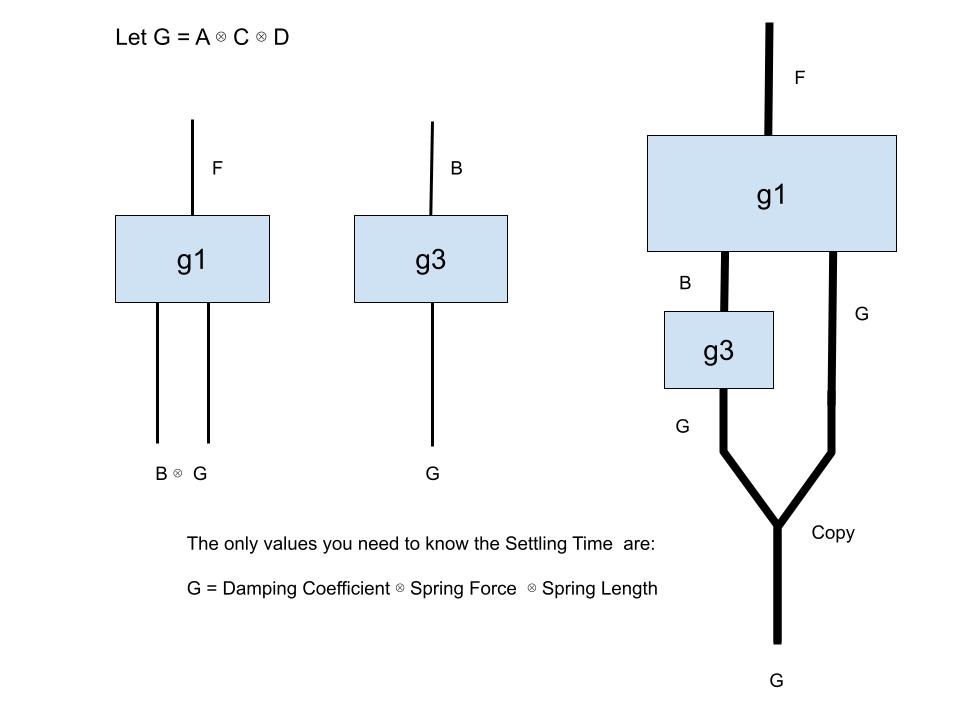top of page
Search
• Ben Sprott

# Dimensionality Reduction With Cavenwell AI

Updated: Sep 11, 2021In this post, we are going to show an interesting application to dimensionality reduction when regressing high dimensional functions. Specifically, we are going to do a little bit of dimensionality reduction in an explainable way.

After running the Cavenwell AI on the data for the damped harmonic oscillator, we found this multi-dimensional function:

g1 = [Damping Coefficient]X[Mass Velocity]X[Spring Force]X[Spring Length]--->Settling Time

We are using the symbol X to represent the cartesian product of columns and this product is actually a monoidal product. Since it is a monoidal product, we are going to use a string diagram to make the process somewhat more explainable.

We start by noting that the AI also found the following multi-dimensional function:

g3 = [Damping Coefficient]X[Spring Force]X[Spring Length]--->Mass Velocity

Some simple software can be created to discover that a smaller set of features can be used, if we simply use two functions instead of one to predict the Settling Time. Here are some diagrams that demonstrates what we mean:Next, we define a smaller dimensional object, namely:

G := [Damping Coefficient]X[Spring Force]X[Spring Length]

We can now plug one function into another to get the following diagram:What this shows is that the lower dimensional object G, can be used to predict the Settling Time, given that one knows how the two functions g3 and g1 can be used.

The AI can explain to the user that she can use a reduced feature set, G, if she regresses the two functions g1 and g3. The AI can also show how this works with the diagram. The Cavenwell AI regresses objects that approximate the functions g3 and g1 and these objects naturally compose as in the string diagram.